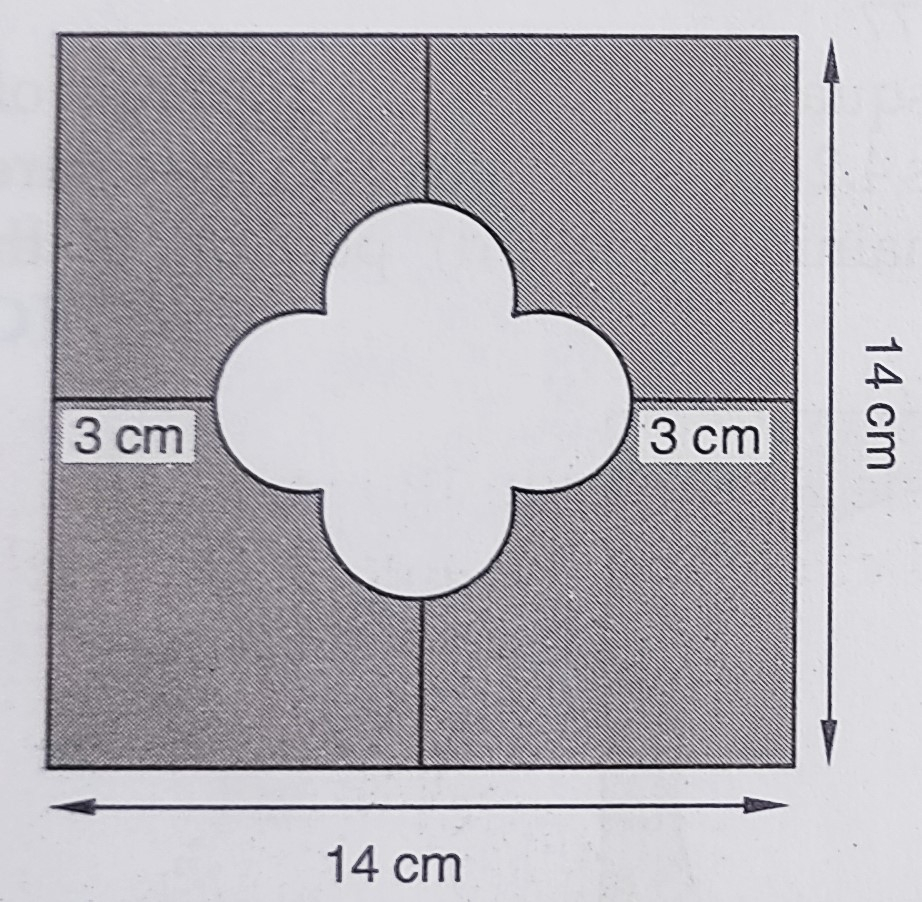"
">

# In the below figure, find the area of the shaded region. (Use $\pi=3.14) ." To do: We have to find the area of the shaded region. Solution: Side of the larger square$= 14\ cm$From the figure,$14=3+3+r+2r+r4r=14-6r=\frac{8}{4}r=2\ cm$This implies, Radius of each semi-circle$= 2\ cm$Side of the inner square$= 4\ cm$Area of the inner square$=  4 \times 4=16\ cm^2$Therefore, Area of four semicircles$= 4 \times \frac{1}{2} \pi r^2= 2 \times 3.14 \times 2^2= 8 \times 3.14= 25.12\ cm^2$Area of the shaded region$=$Area of the large square$-$Area of central part$= (14)^2-(16+ 25.12)\ cm^2= 196-41.12\ cm^2= 154.88\ cm^2$The area of the shaded region is$154.88\ cm^2\$.

Updated on: 10-Oct-2022

183 Views